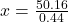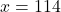## Copy Mark charges $26 + 44 cents per copy to produce promotional brochures a royal linear equation that relates the cost c in dollars of cop Question Copy Mark charges$26 + 44 cents per copy to produce promotional brochures a royal linear equation that relates the cost c in dollars of copies to the number x of copies made and b how many brochures can Steve purchase if he had a budget of $76.16 in progress 0 6 months 2021-08-01T11:14:26+00:00 1 Answers 4 views 0 ## Answers ( ) 1. Answer: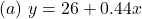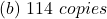Step-by-step explanation: Given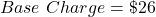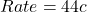per copy Solving (a): Linear Equation The total charges (y) is: the base charge + the rate * number of copies (x). So, we have: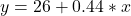The 0.44 is 44 cents converted to dollars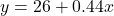Solving (b): Purchase if he budgets$76.16

This implies that y = 76.16

So, we have: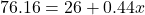Collect like terms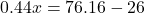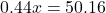Solve for x